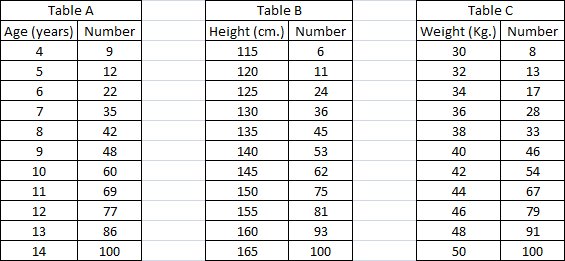### CAT 2003 Question Paper (Leaked) Question 24

Instructions

DIRECTIONS for the following three questions: Answer the questions on the basis of the information given below.Table A below provides data about ages of children in a school. For the age given in the first column, the second column gives the number of children not exceeding the age. For example, first entry indicates that there are 9 children aged 4 years or less. Tables B and C provide data on the heights and weights respectively of the same group of children in a similar format. Assuming that an older child is always taller and weighs more than a younger child, answer the following questions.

Question 24

# How many children of age more than 10 years are taller than 150 cm and do not weigh more than 48 kg?

Solution

There are 40 children of age more than 10 years and 25 children that are taller than 150 cm. Considering 25 which is less than 40 . Also there are 9 children whose weight is more than 48 kg. Hence there are 25-9 = 16 children whose weight will bw less than 48 kg and all other requirement.

• All Quant CAT Formulas and shortcuts PDF
• 30+ CAT previous papers with solutions PDF